# Beginning Machine Learning with Keras & Core ML

In this Keras machine learning tutorial, you’ll learn how to train a convolutional neural network model, convert it to Core ML, and integrate it into an iOS app. By Audrey Tam.

Leave a rating/review
Save for later
Share

## Beginning Machine Learning with Keras & Core ML

50 mins

Apple’s Core ML and Vision frameworks have launched developers into a brave new world of machine learning, with an explosion of exciting possibilities. Vision lets you detect and track faces, and Apple’s Machine Learning page provides ready-to-use models that detect objects and scenes, as well as `NSLinguisticTagger` for natural language processing. If you want to build your own model, try Apple’s new Turi Create to extend one of its pre-trained models with your data.

But if what you want to do needs something even more customized? Then, it’s time to dive into machine learning (ML), using one of the many frameworks from Google, Microsoft, Amazon or Berkeley. And, to make life even more exciting, you’ll need to pick up a new programming language and a new set of development tools.

In this Keras machine learning tutorial you’ll learn how to train a deep-learning convolutional neural network model, convert it to Core ML, and integrate it into an iOS app. You’ll learn some ML terminology, use some new tools, and pick up a bit of Python along the way.

The sample project uses ML’s Hello-World example — a model that classifies hand-written digits, trained on the MNIST dataset.

Let’s get started!

## Why Use Keras?

An ML model involves a lot of complex code, manipulating arrays and matrices. But ML has been around for a long time, and researchers have created libraries that make it much easier for people like us to create ML models. Many of these are written in Python, although researchers also use R, SAS, MATLAB and other software. But you’ll probably find everything you need in the Python-based tools:

• scikit-learn provides an easy way to run many classical ML algorithms, such as linear regression and support vector machines. Our Beginning Machine Learning with scikit-learn tutorial shows you how to train these.
• At the other end of the spectrum are PyTorch and Google’s TensorFlow, which give you greater control over the inner workings of your deep learning model.
• Microsoft’s CNTK and Berkeley’s Caffe are similar deep learning frameworks, which have Python APIs to access their C++ engines.

So where does Keras fit in? It’s a wrapper around TensorFlow and CNTK, with Amazon’s MXNet coming soon. (It also works with Theano, but the University of Montreal stopped working on this in September 2017.) It provides an easy-to-use API for building models that you can train on one backend, and deploy on another.

Another reason to use Keras, rather than directly using TensorFlow, is that `coremltools` includes a Keras converter, but not a TensorFlow converter — although a TensorFlow to CoreML converter and a MXNet to CoreML converter exist. And while Keras supports CNTK as a backend, `coremltools` only works for Keras + TensorFlow.

Note: Do you need to learn Python before you can use these tools? Well, I didn’t ;] As you work through this tutorial, you’ll see that Python syntax is similar to Swift: a bit more streamlined, and indentation is an important part of the syntax. If you’re nervous, keep this open in a browser tab, for quick reference: Jason Brownlee’s Crash Course in Python for Machine Learning Developers.
Another Note: Researchers use both Python 2 and Python 3, but `coremltools` works better with Python 2.7.

## Getting Started

Download and unzip the starter folder: it contains a starter iOS app, where you’ll add the ML model and code to use it. It also contains a docker-keras folder, which contains this tutorial’s Jupyter notebook.

### Setting Up Docker

Docker is a container platform that lets you deploy apps in customized environments — sort of like a virtual machine, but different. Installing Docker gives you access to a large number of ML resources, mostly distributed as interactive Jupyter notebooks in Docker images.

Note: Installing Docker and building the image will take several minutes, so read the ML in a Nutshell section while you wait.

Download, install, and start Docker Community Edition for Mac. In Terminal, enter the following commands, one at a time:

```cd <where you unzipped starter>/starter/docker-keras
docker build -t keras-mnist .
docker run --rm -it -p 8888:8888 -v \$(pwd)/notebook:/workspace/notebook keras-mnist
```

This last command maps the Docker container’s notebook folder to the local notebook folder, so you’ll have access to files written by the notebook, even after you logout of the Docker server.

At the very end of the command output is a URL containing a token. It looks like this, but with a different token value:

```http://0.0.0.0:8888/?token=7b189c8e200f49dcc33845d39101e8a0ab257db5f3b539a7
```

Paste this URL into a browser to login to the Docker container’s notebook server.

Open the notebook folder, then open keras_mnist.ipynb. Tap the Not Trusted button to change it to Trusted: this allows you to save changes you make to the notebook, as well as the model files, in the notebook folder.

### ML in a Nutshell

Arthur Samuel defined machine learning as “the field of study that gives computers the ability to learn without being explicitly programmed”. You have data, which has some features that can be used to classify the data, or use it to make some prediction, but you don’t have an explicit formula for computing this, so you can’t write a program to do it. If you have “enough” data samples, you can train a computer model to recognize patterns in this data, then apply its learning to new data. It’s called supervised learning when you know the correct outcomes for all the training data: then the model just checks its predictions against the known outcomes, and adjusts itself to reduce error and increase accuracy. Unsupervised learning is beyond the scope of this tutorial.

Weights & Threshold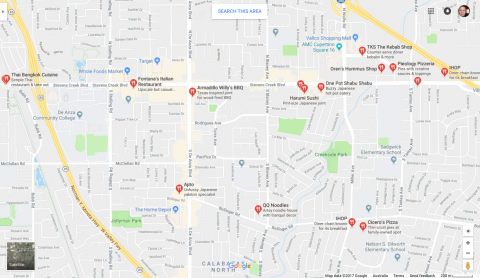Say you want to choose a restaurant for dinner with a group of friends. Several factors influence your decision: dietary restrictions, access to public transport, price range, type of food, child-friendliness, etc. You assign a weight to each factor, to indicate its importance for your decision. Then, for each restaurant in your list of options, you assign a value for each factor, according to how well the restaurant satisfies that factor. You multiply each factor value by the factor’s weight, and add these up to get the weighted sum. The restaurant with the highest result is the best choice. Another way to use this model is to produce binary output: yes or no. You set a threshold value, and remove from your list any restaurant whose weighted sum falls below this threshold.

Training an ML Model

Coming up with the weights isn’t an easy job. But luckily you have a lot of data from previous dinners, including which restaurant was chosen, so you can train an ML model to compute weights that produce the same results, as closely as possible. Then you apply these computed weights to future decisions.

To train an ML model, you start with random weights, apply them to the training data, then compare the computed outputs with the known outputs to calculate the error. This is a multi-dimensional function that has a minimum value, and the goal of training is to determine the weights that get very close to this minimum. The weights also need to work on new data: if the error over a large set of validation data is higher than the error over the training data, then the model is overfitted — the weights work too well on the training data, indicating training has mistakenly detected some feature that doesn’t generalize to new data.

To compute weights that reduce the error, you calculate the gradient of the error function at the current graph location, then adjust the weights to “step down” the slope. This is called gradient descent, and happens many times during a training session. For large datasets, using all the data to calculate the gradient takes a long time. Stochastic gradient descent (SGD) estimates the gradient from randomly selected mini-batches of training data — like taking a survey of voters ahead of election day: if your sample is representative of the whole dataset, then the survey results accurately predict the final results.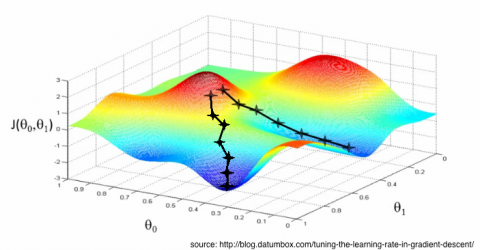Optimizers

The error function is lumpy: you have to be careful not to step too far, or you might miss the minimum. Your step rate also needs to have enough momentum to push you out of any false minimum. ML researchers have put a lot of effort into devising optimization algorithms to do this. The current favorite is Adam (Adaptive Moment estimation), which combines the features of previous favorites RMSprop (Root Mean Square propagation) and AdaGrad (Adaptive Gradient algorithm).

## Keras Code Time!

OK, the Docker container should be ready now: go back and follow the instructions to open the notebook. It’s time to write some Keras code!

Enter the following code in the keras_mnist.ipynb cell with the matching heading. When you finish entering the code in each cell, press Control-Enter to run it. An asterisk appears in the In [ ]: label while the code is running, then a number will appear, to show the order in which you ran the cells. Everything stays in memory while you’re logged in to the notebook. Every so often, tap the Save and Checkpoint button.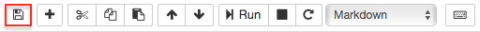Note: Double-click in a markdown cell to add your own comments; press Control-Enter to render the markdown and run your Python code. You can also use the other notebook buttons to add or copy-paste cells, and move cells.

### Import Utilities & Dependencies

Enter the following code, and run it to check the Keras version.

```from __future__ import print_function
from matplotlib import pyplot as plt

import keras
from keras.datasets import mnist
from keras.models import Sequential
from keras.layers import Dense, Dropout, Flatten
from keras.layers import Conv2D, MaxPooling2D
from keras.utils import np_utils
from keras import backend as K

import coremltools
# coremltools supports Keras version 2.0.6
print('keras version ', keras.__version__)
```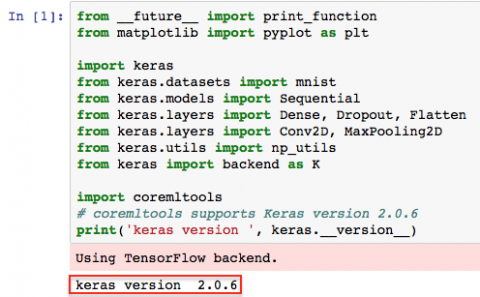`__future__` is the compatibility layer between Python 2 and Python 3: Python 2 has a `print` command (no parentheses), but Python 3 requires a `print()` function. Importing `print_function` allows you to use `print()` statements in Python 2 code.

Keras uses the NumPy mathematics library to manipulate arrays and matrices. Matplotlib is a plotting library for NumPy: you’ll use it to inspect a training data item.

After importing `keras`, print its version: `coremltools` supports version 2.0.6, and will spew warnings if you use a higher version. Keras already has the MNIST dataset, so you import that. Then the next three lines import the model components. You import the NumPy utilities, and you give the backend a label with `import backend as K`: you’ll use it to check `image_data_format`.

Finally, you import `coremltools`, which you’ll use at the end of this notebook.

Training & Validation Data Sets

```(x_train, y_train), (x_val, y_val) = mnist.load_data()
```

This downloads data from https://s3.amazonaws.com/img-datasets/mnist.npz, shuffles the data items, and splits them between a training dataset and a validation dataset. Validation data helps to detect the problem of overfitting the model to the training data. The training step uses the trained parameters to compute outputs for the validation data. You’ll set callbacks to monitor validation loss and accuracy, to save the model that performs best on the validation data, and possibly stop early, if validation loss or accuracy fail to improve for too many epochs (repetitions).

Inspect x & y Data

When the download finishes, enter the following code in the next cell, and run it to see what you got.

Note: You don’t have to enter the lines beginning with `#`. These are comments, and most of them are here to show you what the notebook should display when you run the cell.
```# Inspect x data
print('x_train shape: ', x_train.shape)
# Displays (60000, 28, 28)
print(x_train.shape, 'training samples')
# Displays 60000 train samples
print('x_val shape: ', x_val.shape)
# Displays (10000, 28, 28)
print(x_val.shape, 'validation samples')
# Displays 10000 validation samples

print('First x sample\n', x_train)
# Displays an array of 28 arrays, each containing 28 gray-scale values between 0 and 255
# Plot first x sample
plt.imshow(x_train)
plt.show()

# Inspect y data
print('y_train shape: ', y_train.shape)
# Displays (60000,)
print('First 10 y_train elements:', y_train[:10])
# Displays [5 0 4 1 9 2 1 3 1 4]
```

You have 60,000 28×28-pixel training samples and 10,000 validation samples. The first training sample is an array of 28 arrays, each containing 28 gray-scale values between 0 and 255. Looking at the non-zero values, you can see a shape like the digit 5.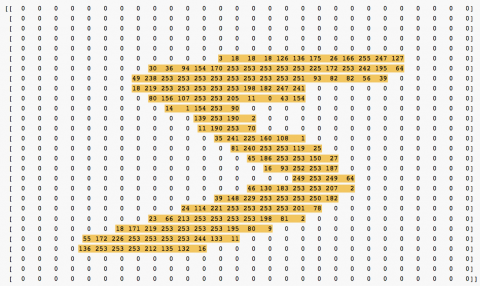Sure enough, the `plt` code shows the first training sample is a handwritten 5: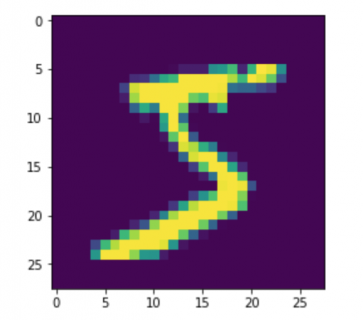The y data is a 60000-element array containing the correct classifications of the training samples: the first training sample is 5, the next is 0, and so on.

Set Input & Output Dimensions

Enter these two lines, and run the cell to set up the basic dimensions of the x inputs and y outputs.

```img_rows, img_cols = x_train.shape, x_train.shape
num_classes = 10
```

MNIST data items are 28×28-pixel images, and you want to classify each as a digit between 0 and 9.

You use `x_train.shape` values to set the number of image rows and columns. `x_train.shape` is an array of 3 elements:

1. number of data samples: 60000
2. number of rows of each data sample: 28
3. number of columns of each data sample: 28

Reshape x Data & Set Input Shape

The model needs the data in a slightly different “shape”. Enter the code below, and run it.

```# Set input_shape for channels_first or channels_last
if K.image_data_format() == 'channels_first':
x_train = x_train.reshape(x_train.shape, 1, img_rows, img_cols)
x_val = x_val.reshape(x_val.shape, 1, img_rows, img_cols)
input_shape = (1, img_rows, img_cols)
else:
x_train = x_train.reshape(x_train.shape, img_rows, img_cols, 1)
x_val = x_val.reshape(x_val.shape, img_rows, img_cols, 1)
input_shape = (img_rows, img_cols, 1)
```

Convolutional neural networks think of images as having width, height and depth. The depth dimension is called channels, and contains color information. Gray-scale images have 1 channel; RGB images have 3 channels.

Keras backends like TensorFlow and CNTK, expect image data in either channels-last format (rows, columns, channels) or channels-first format (channels, rows, columns). The `reshape` function inserts the channels in the correct position.

You also set the initial `input_shape` with the channels at the correct end.

Inspect Reshaped x Data

Enter the code below, and run it to see how the shapes have changed.

```print('x_train shape:', x_train.shape)
# x_train shape: (60000, 28, 28, 1)
print('x_val shape:', x_val.shape)
# x_val shape: (10000, 28, 28, 1)
print('input_shape:', input_shape)
# input_shape: (28, 28, 1)
```

TensorFlow image data format is channels-last, so `x_train.shape` and `x_val.shape` now have a new element, 1, at the end.

Convert Data Type & Normalize Values

The model needs the data values in a specific format. Enter the code below, and run it.

```x_train = x_train.astype('float32')
x_val = x_val.astype('float32')
x_train /= 255
x_val /= 255
```

MNIST image data values are of type `uint8`, in the range [0, 255], but Keras needs values of type `float32`, in the range [0, 1].

Inspect Normalized x Data

Enter the code below, and run it to see the changes to the x data.

```print('First x sample, normalized\n', x_train)
# An array of 28 arrays, each containing 28 arrays, each with one value between 0 and 1
```

Now each value is an array, the values are floats, and the non-zero values are between 0 and 1.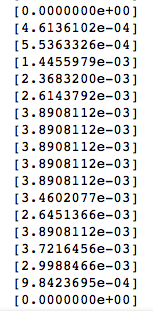Reformat y Data

The y data is a 60000-element array containing the correct classifications of the training samples, but it’s not obvious that there are only 10 categories. Enter the code below, and run it once only to reformat the y data.

```print('y_train shape: ', y_train.shape)
# (60000,)
print('First 10 y_train elements:', y_train[:10])
# [5 0 4 1 9 2 1 3 1 4]
# Convert 1-dimensional class arrays to 10-dimensional class matrices
y_train = np_utils.to_categorical(y_train, num_classes)
y_val = np_utils.to_categorical(y_val, num_classes)
print('New y_train shape: ', y_train.shape)
# (60000, 10)
```

`y_train` is a 1-dimensional array, but the model needs a 60000 x 10 matrix to represent the 10 categories. You must also make the same conversion for the 10000-element `y_val` array.

Inspect Reformatted y Data

Enter the code below, and run it to see how the y data has changed.

```print('New y_train shape: ', y_train.shape)
# (60000, 10)
print('First 10 y_train elements, reshaped:\n', y_train[:10])
# An array of 10 arrays, each with 10 elements,
# all zeros except at index 5, 0, 4, 1, 9 etc.
```

`y_train` is now an array of 10-element arrays, each containing all zeros except at the index that the image matches.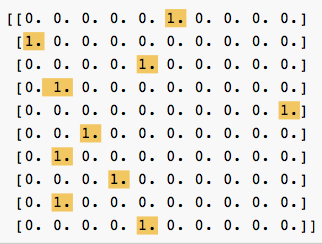### Define Model ArchitectureModel architecture is a form of alchemy, like secret family recipes for the perfect barbecue sauce or garam masala. You might start with a general-purpose architecture, then tweak it to exploit symmetries in your input data, or to produce a model with specific characteristics.

Here are models from two researchers: Sri Raghu Malireddi and François Chollet, the author of Keras. Chollet’s is general-purpose, and Malireddi’s is designed to produce a small model, suitable for mobile apps.

Enter the code below, and run it to see the model summaries.

Malireddi’s Architecture

```model_m = Sequential()
# Inspect model's layers, output shapes, number of trainable parameters
print(model_m.summary())
```

Chollet’s Architecture

```model_c = Sequential()
# Note: hwchong, elitedatascience use 32 for second Conv2D
# Inspect model's layers, output shapes, number of trainable parameters
print(model_c.summary())
```

Although Malireddi’s architecture has one more convolutional layer (`Conv2D`) than Chollet’s, it runs much faster, and the resulting model is much smaller.

Model Summaries

Take a quick look at the model summaries for these two models:

model_m:

```Layer (type)                 Output Shape              Param #
=================================================================
conv2d_6 (Conv2D)            (None, 24, 24, 32)        832
_________________________________________________________________
max_pooling2d_5 (MaxPooling2 (None, 12, 12, 32)        0
_________________________________________________________________
dropout_6 (Dropout)          (None, 12, 12, 32)        0
_________________________________________________________________
conv2d_7 (Conv2D)            (None, 10, 10, 64)        18496
_________________________________________________________________
max_pooling2d_6 (MaxPooling2 (None, 5, 5, 64)          0
_________________________________________________________________
dropout_7 (Dropout)          (None, 5, 5, 64)          0
_________________________________________________________________
conv2d_8 (Conv2D)            (None, 5, 5, 128)         8320
_________________________________________________________________
max_pooling2d_7 (MaxPooling2 (None, 2, 2, 128)         0
_________________________________________________________________
dropout_8 (Dropout)          (None, 2, 2, 128)         0
_________________________________________________________________
flatten_3 (Flatten)          (None, 512)               0
_________________________________________________________________
dense_5 (Dense)              (None, 128)               65664
_________________________________________________________________
dense_6 (Dense)              (None, 10)                1290
=================================================================
Total params: 94,602
Trainable params: 94,602
Non-trainable params: 0
```

model_c:

```Layer (type)                 Output Shape              Param #
=================================================================
conv2d_4 (Conv2D)            (None, 26, 26, 32)        320
_________________________________________________________________
conv2d_5 (Conv2D)            (None, 24, 24, 64)        18496
_________________________________________________________________
max_pooling2d_4 (MaxPooling2 (None, 12, 12, 64)        0
_________________________________________________________________
dropout_4 (Dropout)          (None, 12, 12, 64)        0
_________________________________________________________________
flatten_2 (Flatten)          (None, 9216)              0
_________________________________________________________________
dense_3 (Dense)              (None, 128)               1179776
_________________________________________________________________
dropout_5 (Dropout)          (None, 128)               0
_________________________________________________________________
dense_4 (Dense)              (None, 10)                1290
=================================================================
Total params: 1,199,882
Trainable params: 1,199,882
Non-trainable params: 0
```

The bottom line Total params is the main reason for the size difference: Chollet’s 1,199,882 is 12.5 times more than Malireddi’s 94,602. And that’s just about exactly the difference in model size: 4.8MB vs 380KB.

Malireddi’s model has three `Conv2D` layers, each followed by a `MaxPooling2D` layer, which halves the layer’s width and height. This makes the number of parameters for the first dense layer much smaller than Chollet’s, and explains why Malireddi’s model is much smaller and trains much faster. The implementation of convolutional layers is highly optimized, so the additional convolutional layer improves the accuracy without adding much to training time. But the smaller dense layer runs much faster than Chollet’s.

I’ll tell you about layers, output shape and parameter numbers in the Explanations section, while you wait for the next step to finish running.

### Train the Model

Define Callbacks List

`callbacks` is an optional argument for the `fit` function, so define `callbacks_list` first.

Enter the code below, and run it.

```callbacks_list = [
keras.callbacks.ModelCheckpoint(
filepath='best_model.{epoch:02d}-{val_loss:.2f}.h5',
monitor='val_loss', save_best_only=True),
keras.callbacks.EarlyStopping(monitor='acc', patience=1)
]
```

An epoch is a complete pass through all the mini-batches in the dataset.

The `ModelCheckpoint` callback monitors the validation loss value, saving the model with the lowest-so-far value in a file with the epoch number and the validation loss in the filename.

The `EarlyStopping` callback monitors training accuracy: if it fails to improve for two consecutive epochs, training stops early. In my experiments, this never happened: if `acc` went down in one epoch, it always recovered in the next.

Compile & Fit Model

Unless you have access to a GPU, I recommend you use Malireddi’s `model_m` for this step, as it runs much faster than Chollet’s `model_c`: on my MacBook Pro, 76-106s/epoch vs. 246-309s/epoch, or about 15 minutes vs. 45 minutes.

Note: If an .h5 file doesn’t appear in notebook after the first epoch finishes, click the stop button to interrupt the kernel, click the save button, and logout. In Terminal, press Control-C to stop the server, then re-run the `docker run` command. Paste the URL or token into the browser or login page, navigate to the notebook, and click the Not Trusted button. Select this cell, then select Cell\Run All Above from the menu.

Enter the code below, and run it. This will take quite a while, so read the Explanations section while you wait. But check Finder after a couple of minutes, to make sure the notebook is saving .h5 files.

Note: This cell shows the two types of indentation for multi-line function calls, depending on where you write the first argument. It’s a syntax error if it’s out by even one space.
```model_m.compile(loss='categorical_crossentropy',

# Hyper-parameters
batch_size = 200
epochs = 10

# Enable validation to use ModelCheckpoint and EarlyStopping callbacks.
model_m.fit(
x_train, y_train, batch_size=batch_size, epochs=epochs,
callbacks=callbacks_list, validation_data=(x_val, y_val), verbose=1)
```

## Convolutional Neural Network: Explanations

You can use just about any ML approach to create an MNIST classifier, but this tutorial uses a convolutional neural network (CNN), because that’s a key strength of TensorFlow and Keras.

Convolutional neural networks assume inputs are images, and arrange neurons in three dimensions: width, height, depth. A CNN consists of convolutional layers, each detecting higher-level features of the training images: the first layer might train filters to detect short lines or arcs at various angles; the second layer trains filters to detect significant combinations of these lines; the final layer’s filters build on the previous layers to classify the image.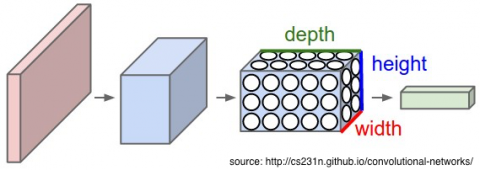Each convolutional layer passes a small square kernel of weights — 1×1, 3×3 or 5×5 — over the input, computing the weighted sum of the input units under the kernel. This is the convolution process.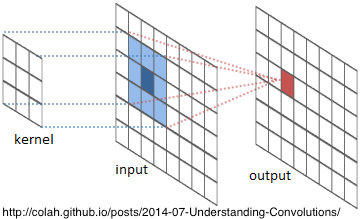Each neuron is connected to only 1, 9, or 25 neurons in the previous layer, so there’s a danger of co-adapting — depending too much on a few inputs — and this can lead to overfitting. So CNNs include pooling and dropout layers to counteract co-adapting and overfitting. I explain these, below.

Sample Model

Here’s Malireddi’s model again:

```model_m = Sequential()
```

Let’s work our way through this code.

### Sequential

You first create an empty `Sequential` model, then add a linear stack of layers: the layers run in the sequence that they’re added to the model. The Keras documentation has several examples of `Sequential` models.

Note: Keras also has a functional API for defining complex models, such as multi-output models, directed acyclic graphs, or models with shared layers. Google’s Inception and Microsoft Research Asia’s Residual Networks are examples of complex models with nonlinear connectivity structures.

The first layer must have information about the input shape, which for MNIST is (28, 28, 1). The other layers infer their input shape from the output shape of the previous layer. Here’s the output shape part of the model summary:

```Layer (type)                 Output Shape              Param #
=================================================================
conv2d_6 (Conv2D)            (None, 24, 24, 32)        832
_________________________________________________________________
max_pooling2d_5 (MaxPooling2 (None, 12, 12, 32)        0
_________________________________________________________________
dropout_6 (Dropout)          (None, 12, 12, 32)        0
_________________________________________________________________
conv2d_7 (Conv2D)            (None, 10, 10, 64)        18496
_________________________________________________________________
max_pooling2d_6 (MaxPooling2 (None, 5, 5, 64)          0
_________________________________________________________________
dropout_7 (Dropout)          (None, 5, 5, 64)          0
_________________________________________________________________
conv2d_8 (Conv2D)            (None, 5, 5, 128)         8320
_________________________________________________________________
max_pooling2d_7 (MaxPooling2 (None, 2, 2, 128)         0
_________________________________________________________________
dropout_8 (Dropout)          (None, 2, 2, 128)         0
_________________________________________________________________
flatten_3 (Flatten)          (None, 512)               0
_________________________________________________________________
dense_5 (Dense)              (None, 128)               65664
_________________________________________________________________
dense_6 (Dense)              (None, 10)                1290
```

### Conv2D

This model has three `Conv2D` layers:

```Conv2D(32, (5, 5), input_shape=input_shape, activation='relu')
Conv2D(64, (3, 3), activation='relu')
Conv2D(128, (1, 1), activation='relu')
```
• The first parameter — 32, 64, 128 — is the number of filters, or features, you want to train this layer to detect. This is also the depth — the last dimension — of the output shape.
• The second parameter — (5, 5), (3, 3), (1, 1) — is the kernel size: a tuple specifying the width and height of the convolution window that slides over the input space, computing weighted sums — dot products of the kernel weights and the input unit values.
• The third parameter `activation='relu'` specifies the ReLU (Rectified Linear Unit) activation function. When the kernel is centered on an input unit, the unit is said to activate or fire if the weighted sum is greater than a threshold value: `weighted_sum > threshold`. The `bias` value is `-threshold`: the unit fires if `weighted_sum + bias > 0`. Training the model calculates the kernel weights and the bias value for each filter. ReLU is the most popular activation function for deep neural networks.

### MaxPooling2D

```MaxPooling2D(pool_size=(2, 2))
```

A pooling layer slides an n-rows by m-columns filter across the previous layer, replacing the n x m values with their maximum value. Pooling filters are usually square: n = m. The most commonly used 2 x 2 pooling filter, shown below, halves the width and height of the previous layer, thus reducing the number of parameters, which helps control overfitting.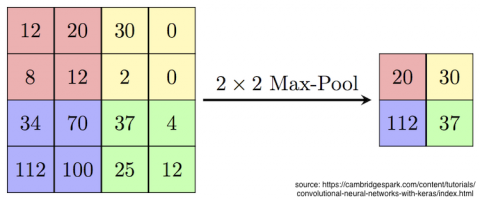Malireddi’s model has a pooling layer after each convolutional layer, which greatly reduces the final model size and training time.

Chollet’s model has two convolutional layers before pooling. This is recommended for larger networks, as it allows the convolutional layers to develop more complex features before pooling discards 75% of the values.

`Conv2D` and `MaxPooling2D` parameters determine each layer’s output shape and number of trainable parameters:

Output Shape = (input width – kernel width + 1, input height – kernel height + 1, number of filters)

You can’t center a 3×3 kernel over the first and last units in each row and column, so the output width and height are 2 pixels less than the input. A 5×5 kernel reduces output width and height by 4 pixels.

• `Conv2D(32, (5, 5), input_shape=(28, 28, 1))`: (28-4, 28-4, 32) = (24, 24, 32)
• `MaxPooling2D` halves the input width and height: (24/2, 24/2, 32) = (12, 12, 32)
• `Conv2D(64, (3, 3))`: (12-2, 12-2, 64) = (10, 10, 64)
• `MaxPooling2D` halves the input width and height: (10/2, 10/2, 64) = (5, 5, 64)
• `Conv2D(128, (1, 1))`: (5-0, 5-0, 128) = (5, 5, 128)

Param # = number of filters x (kernel width x kernel height x input depth + 1 bias)

• `Conv2D(32, (5, 5), input_shape=(28, 28, 1))`: 32 x (5x5x1 + 1) = 832
• `Conv2D(64, (3, 3))`: 64 x (3x3x32 + 1) = 18,496
• `Conv2D(128, (1, 1))`: 128 x (1x1x64 + 1) = 8320

Challenge: Calculate the output shapes and parameter numbers for Chollet’s architecture `model_c`.

[spoiler title=”Solution”]
Output Shape = (input width – kernel width + 1, input height – kernel height + 1, number of filters)

• `Conv2D(32, (3, 3), input_shape=(28, 28, 1))`: (28-2, 28-2, 32) = (26, 26, 32)
• `Conv2D(64, (3, 3))`: (26-2, 26-2, 64) = (24, 24, 64)
• `MaxPooling2D` halves the input width and height: (24/2, 24/2, 64) = (12, 12, 64)

Param # = number of filters x (kernel width x kernel height x input depth + 1 bias)

• `Conv2D(32, (3, 3), input_shape=(28, 28, 1))`: 32 x (3x3x1 + 1) = 320
• `Conv2D(64, (3, 3))`: 64 x (3x3x32 + 1) = 18,496

[/spoiler]

### Dropout

```Dropout(0.5)
Dropout(0.2)
```

A dropout layer is often paired with a pooling layer. It randomly sets a fraction of input units to 0. This is another method to control overfitting: neurons are less likely to be influenced too much by neighboring neurons, because any of them might drop out of the network at random. This makes the network less sensitive to small variations in the input, so more likely to generalize to new inputs.

Aurélien Géron, in Hands-on Machine Learning with Scikit-Learn & TensorFlow, compares this to a workplace where, on any given day, some percentage of the people might not come to work: everyone would have to be able to do critical tasks, and would have to cooperate with more co-workers. This would make the company more resilient, and less dependent on any single worker.

### Flatten

The weights from the convolutional layers must be made 1-dimensional — flattened — before passing them to the fully connected Dense layer.

```model_m.add(Dropout(0.2))
```

The output shape of the previous layer is (2, 2, 128), so the output of `Flatten()` is an array with 512 elements.

### Dense

```Dense(128, activation='relu')
Dense(num_classes, activation='softmax')
```

Each neuron in a convolutional layer uses the values of only a few neurons in the previous layer. Each neuron in a fully connected layer uses the values of all the neurons in the previous layer. The Keras name for this type of layer is `Dense`.

Looking at the model summaries above, Malireddi’s first `Dense` layer has 512 neurons, while Chollet’s has 9216. Both produce a 128-neuron output layer, but Chollet’s must compute 18 times more parameters than Malireddi’s. This is what uses most of the additional training time.

Most CNN architectures end with one or more `Dense` layers and then the output layer.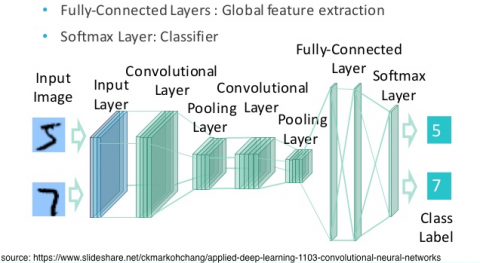The first parameter is the output size of the layer. The final output layer has an output size of 10, corresponding to the 10 classes of digits.

The `softmax` activation function produces a probability distribution over the 10 output classes. It’s a generalization of the sigmoid function, which scales its input value into the range [0, 1]. For your MNIST classifier, `softmax` scales each of 10 values into [0, 1], such that they add up to 1.

You would use the sigmoid function for a single output class: for example, what’s the probability that this is a photo of a good dog?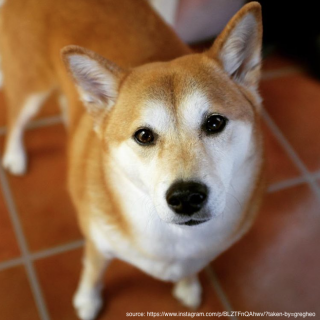### Compile

```model_m.compile(loss='categorical_crossentropy', optimizer='adam', metrics=['accuracy'])
```

The categorical crossentropy loss function measures the distance between the probability distribution calculated by the CNN, and the true distribution of the labels.

An optimizer is the stochastic gradient descent algorithm that tries to minimize the loss function by following the gradient down at just the right speed.

Accuracy — the fraction of the images that were correctly classified — is the most common metric monitored during training and testing.

### Fit

```batch_size = 256
epochs = 10
model_m.fit(x_train, y_train, batch_size=batch_size, epochs=epochs, callbacks=callbacks_list,
validation_data=(x_val, y_val), verbose=1)
```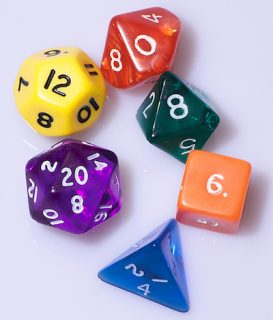Batch size is the number of data items to use for mini-batch stochastic gradient fitting. Choosing a batch size is a matter of trial and error, a roll of the dice. Smaller values make epochs take longer; larger values make better use of GPU parallelism, and reduce data transfer time, but too large might cause you to run out of memory.

The number of epochs is also a roll of the dice. Each epoch should improve loss and accuracy measurements. More epochs should produce a more accurate model, but training takes longer. Too many epochs can result in overfitting. You set up a callback to stop early, if the model stops improving before completing all the epochs. In the notebook, you can re-run the `fit` cell to keep improving the model.

When you loaded the data, 10000 items were set as validation data. Passing this argument enables validation while training, so you can monitor validation loss and accuracy. If these values are worse than the training loss and accuracy, this indicates that the model is overfitted.

### Verbose

0 = silent, 1 = progress bar, 2 = one line per epoch.

## Results

Here’s the result of one of my training runs:

```Epoch 1/10
60000/60000 [==============================] - 106s - loss: 0.0284 - acc: 0.9909 - val_loss: 0.0216 - val_acc: 0.9940
Epoch 2/10
60000/60000 [==============================] - 100s - loss: 0.0271 - acc: 0.9911 - val_loss: 0.0199 - val_acc: 0.9942
Epoch 3/10
60000/60000 [==============================] - 102s - loss: 0.0260 - acc: 0.9914 - val_loss: 0.0228 - val_acc: 0.9931
Epoch 4/10
60000/60000 [==============================] - 101s - loss: 0.0257 - acc: 0.9913 - val_loss: 0.0211 - val_acc: 0.9935
Epoch 5/10
60000/60000 [==============================] - 101s - loss: 0.0256 - acc: 0.9916 - val_loss: 0.0222 - val_acc: 0.9928
Epoch 6/10
60000/60000 [==============================] - 100s - loss: 0.0263 - acc: 0.9913 - val_loss: 0.0178 - val_acc: 0.9950
Epoch 7/10
60000/60000 [==============================] - 87s - loss: 0.0231 - acc: 0.9920 - val_loss: 0.0212 - val_acc: 0.9932
Epoch 8/10
60000/60000 [==============================] - 76s - loss: 0.0240 - acc: 0.9922 - val_loss: 0.0212 - val_acc: 0.9935
Epoch 9/10
60000/60000 [==============================] - 76s - loss: 0.0261 - acc: 0.9916 - val_loss: 0.0220 - val_acc: 0.9934
Epoch 10/10
60000/60000 [==============================] - 76s - loss: 0.0231 - acc: 0.9925 - val_loss: 0.0203 - val_acc: 0.9935
```

With each epoch, loss values should decrease, and accuracy values should increase. The `ModelCheckpoint` callback saves epochs 1, 2 and 6, because validation loss values in epochs 3, 4 and 5 are higher than epoch 2’s, and there’s no improvement in validation loss after epoch 6. Training doesn’t stop early, because training accuracy never decreases for two consecutive epochs.

Note: Actually, these results are from 20 or 30 epochs: I ran the `fit` cell more than once, without resetting the model, so loss and accuracy values are already quite good, even in epoch 1. But you see some wavering in the measurements, for example, accuracy decreases in epochs 4, 6 and 9.

By now, your model has finished training, so back to coding!

## Convert to Core ML Model

When the training step is complete, you should have a few models saved in notebook. The one with the highest epoch number (and lowest validation loss) is the best model, so use that filename in the `convert` function.

Enter the following code, and run it.

```output_labels = ['0', '1', '2', '3', '4', '5', '6', '7', '8', '9']
# For the first argument, use the filename of the newest .h5 file in the notebook folder.
coreml_mnist = coremltools.converters.keras.convert(
'best_model.09-0.03.h5', input_names=['image'], output_names=['output'],
class_labels=output_labels, image_input_names='image')
```

Here, you set the 10 output labels in an array, and pass this as the `class_labels` argument. If you train a model with a lot of output classes, put the labels in a text file, one label per line, and set the `class_labels` argument to the file name.

In the parameter list, you supply input and output names, and set `image_input_names='image'` so the Core ML model accepts an image as input, instead of a multi-array.

### Inspect Core ML model

Enter this line, and run it to see the printout.

```print(coreml_mnist)
```

Just check that the input type is `imageType`, not multi-array:

```input {
name: "image"
shortDescription: "Digit image"
type {
imageType {
width: 28
height: 28
colorSpace: GRAYSCALE
}
}
}
```

Now add the following, substituting your own name and license info for the first two items, and run it.

```coreml_mnist.author = 'raywenderlich.com'
coreml_mnist.short_description = 'Image based digit recognition (MNIST)'
coreml_mnist.input_description['image'] = 'Digit image'
coreml_mnist.output_description['output'] = 'Probability of each digit'
coreml_mnist.output_description['classLabel'] = 'Labels of digits'
```

This information appears when you select the model in Xcode’s Project navigator.

### Save the Core ML Model

Finally, add the following, and run it.

```coreml_mnist.save('MNISTClassifier.mlmodel')
```

This saves the mlmodel file in the notebook folder.

Congratulations, you now have a Core ML model that classifies handwritten digits! It’s time to use it in the iOS app.## Use Model in iOS App

Now you just follow the procedure described in Core ML and Vision: Machine Learning in iOS 11 Tutorial. The steps are the same, but I’ve rearranged the code to match Apple’s sample app Image Classification with Vision and CoreML.

Step 1. Drag the model into the app:

Open the starter app in Xcode, and drag MNISTClassifier.mlmodel from Finder into the project’s Project navigator. Select it to see the metadata you added: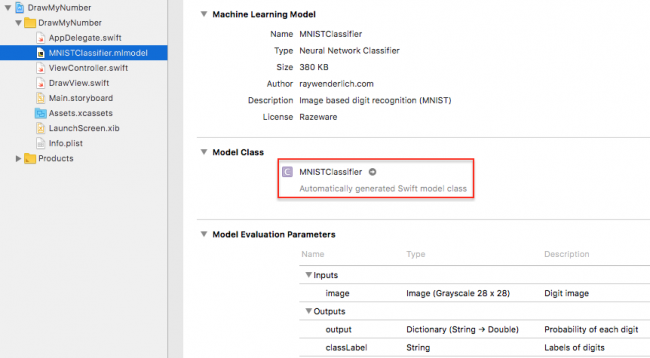If instead of Automatically generated Swift model class it says to build the project to generate the model class, go ahead and do that.

Step 2. Import the `CoreML` and `Vision` frameworks:

Open ViewController.swift, and import the two frameworks, just below `import UIKit`:

```import CoreML
import Vision
```

Step 3. Create `VNCoreMLModel` and `VNCoreMLRequest` objects:

Add the following code below the outlets:

```lazy var classificationRequest: VNCoreMLRequest = {
// Load the ML model through its generated class and create a Vision request for it.
do {
let model = try VNCoreMLModel(for: MNISTClassifier().model)
return VNCoreMLRequest(model: model, completionHandler: handleClassification)
} catch {
fatalError("Can't load Vision ML model: \(error).")
}
}()

func handleClassification(request: VNRequest, error: Error?) {
guard let observations = request.results as? [VNClassificationObservation]
else { fatalError("Unexpected result type from VNCoreMLRequest.") }
guard let best = observations.first
else { fatalError("Can't get best result.") }

DispatchQueue.main.async {
self.predictLabel.text = best.identifier
self.predictLabel.isHidden = false
}
}
```

The request object works for any image that the handler in Step 4 passes to it, so you only need to define it once, as a `lazy var`.

The request object’s completion handler receives `request` and `error` objects. You check that `request.results` is an array of `VNClassificationObservation` objects, which is what the Vision framework returns when the Core ML model is a classifier, rather than a predictor or image processor.

A `VNClassificationObservation` object has two properties: `identifier` — a `String` — and `confidence` — a number between 0 and 1 — the probability the classification is correct. You take the first result, which will have the highest confidence value, and dispatch back to the main queue to update `predictLabel`. Classification work happens off the main queue, because it can be slow.

Step 4. Create and run a `VNImageRequestHandler`:

Locate `predictTapped()`, and replace the `print` statement with the following code:

```let ciImage = CIImage(cgImage: inputImage)
let handler = VNImageRequestHandler(ciImage: ciImage)
do {
try handler.perform([classificationRequest])
} catch {
print(error)
}
```

You create a `CIImage` from `inputImage`, then create the `VNImageRequestHandler` object for this `ciImage`, and run the handler on an array of `VNCoreMLRequest` objects — in this case, just the one request object you created in Step 3.

Build and run. Draw a digit in the center of the drawing area, then tap Predict. Tap Clear to try again.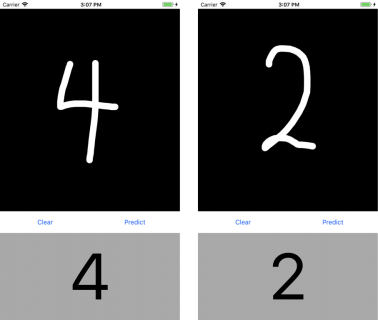Larger drawings tend to work better, but the model often has trouble with ‘7’ and ‘4’. Not surprising, as a PCA visualization of the MNIST data shows 7s and 4s clustered with 9s: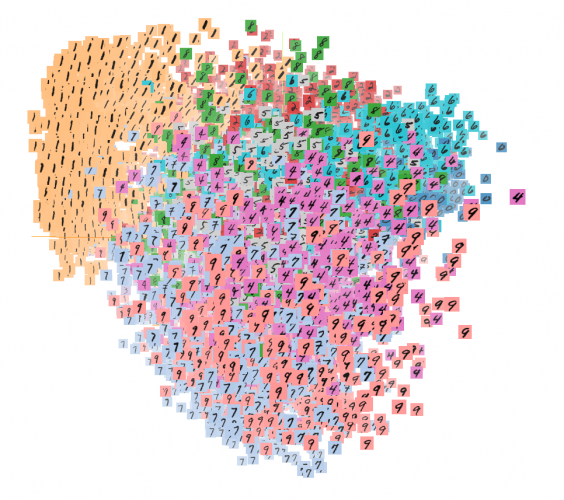Note: Malireddi says the Vision framework uses 20% more CPU, so his app includes an extension to convert a `UIImage` object to `CVPixelBuffer` format.

If you don’t use Vision, include `image_scale=1/255.0` as a parameter when you convert the Keras model to Core ML: the Keras model trains on images with gray scale values in the range [0, 1], and `CVPixelBuffer` values are in the range [0, 255].

Thanks to Sri Raghu M, Matthijs Hollemans and Hon Weng Chong for helpful discussions!

## Where To Go From Here?

You can download the complete notebook and project for this tutorial here. If the model shows up as missing in the app, replace it with the one in the notebook folder.

You’re now well-equipped to train a deep learning model in Keras, and integrate it into your app. Here are some resources and further reading to deepen your own learning:

### ResourcesAudrey Tam